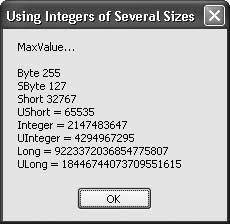# Recipe 6.2. Choosing Integers of the Right Size and Type for the Job

#### Problem

You want to use the right- sized integer variable for the job at hand.

#### Solution

Sample code folder: Chapter 06\UsingIntegers

Visual Basic 2005 now has signed and unsigned integer variable types that range in size from 8 bits to 64 bits (1 byte to 8 bytes). Using the right size and type of integer can save memory, generate more efficient code, and provide ranges of integer values suitable to a variety of needs.

#### Discussion

Visual Basic 2005 is the first version of Visual Basic to support signed byte values and unsigned integer values in a variety of sizes. Here's a list of all the integer types now supported:

Byte

Eight-bit (1-byte) values ranging from 0 to 255. Equivalent to System.Byte.

SByte

A signed type that is 8 bits (1 byte) in size and holds values ranging from -128 to +127. Equivalent to System.SByte.

Short

Sixteen-bit (2-byte) values ranging from -32,768 to +32,767. Equivalent to System.Int16.

UInt16

An unsigned type that is 16 bits (2 bytes) in size and holds values ranging from 0 to 65,535. Equivalent to System.UInt16.

Integer

Thirty-two-bit (4-byte) values ranging from -2,147,483,648 to +2,147,483,647. Equivalent to System.Int32.

UInteger

An unsigned type that is 32 bits (4 bytes) in size and holds values ranging from 0 to 4,294,967,295. Equivalent to System.UInt32.

Long

Sixty-four-bit (8-byte) values ranging from -9,223,372,036,854,775,808 to +9,223,372,036,854,775,807 (-9 to +9 quintillion). Equivalent to System.Int64.

ULong

An unsigned type that is 64 bits (8 bytes) in size and holds values ranging from 0 to 18,446,744,073,709,551,615 (18 quintillion). Equivalent to System.UInt64.

The following code demonstrates each of these integer types by displaying the largest possible value for each:

` Dim result As New System.Text.StringBuilder() result.AppendLine("MaxValue…") result.AppendLine() Dim maxByte As Byte = Byte.MaxValue Dim maxSByte As SByte = SByte.MaxValue Dim maxShort As Short = Short.MaxValue Dim maxUShort As UShort = UShort.MaxValue Dim maxInteger As Integer = Integer.MaxValue Dim maxUInteger As UInteger = UInteger.MaxValue Dim maxLong As Long = Long.MaxValue Dim maxULong As ULong = ULong.MaxValue result.Append("Byte ").AppendLine(maxByte) result.Append("SByte ").AppendLine(maxSByte) result.Append("Short ").AppendLine(maxShort) result.Append("UShort = ").AppendLine(maxUShort) result.Append("Integer = ").AppendLine(maxInteger) result.Append("UInteger = ").AppendLine(maxUInteger) result.Append("Long = ").AppendLine(maxLong) result.Append("ULong = ").AppendLine(maxULong) MsgBox(result.ToString()) `

For all unsigned variable types, the minimum possible value is zero. For all signed types, to find the minimum value add one to the maximum value, and change the sign. For example, the maximum value for signed bytes is 127, and the minimum value is -128. As shown above, the MaxValue property of each integer type provides a straightforward way to access the largest possible value. Similarly, you can get the smallest possible value by accessing each type's MinValue property.

Figure 6-2 shows the maximum values for each type of integer, as displayed by the message box in the example code.

##### Figure 6-2. Maximum values for the various integer variable typesOne other variable type is worth considering for extremely large integer values. Although not true integers, Decimal variables can hold integer values up to 79,228,162,514,264,337,593,543,950,335 (79 octillion). The rule for determining the minimum value for a Decimal-type variable is slightly different than for the true integer types: in this case, just reverse the sign of the maximum value, don't add 1. The MinValue for Decimal variables is thus -79,228,162,514,264,337,593,543,950,335.

Decimal values are signed 128-bit (16-byte) numbers, and they may have a decimal point. If you appropriately round off or truncate values, the Decimal type can accurately hold extremely large integer values. However, even on 64-bit machines, this data type can slow down calculations somewhat because the processor must perform calculations using multiple steps to process each value.Visual Basic 2005 Cookbook: Solutions for VB 2005 Programmers (Cookbooks (OReilly))
ISBN: 0596101775
EAN: 2147483647
Year: 2006
Pages: 400

Similar book on Amazon